Chapter 3: Graphing

# Finding the Distance Between Two Points

The logic used to find the distance between two data points on a graph involves the construction of a right triangle using the two data points and the Pythagorean theorem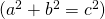to find the distance.

To do this for the two data points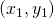and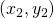, the distance between these two points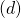will be found using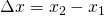and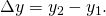Using the Pythagorean theorem, this will end up looking like: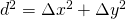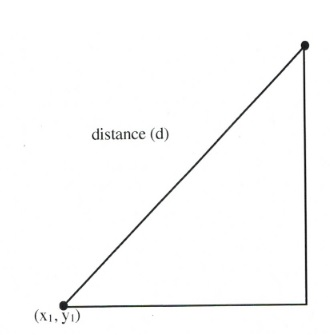or, in expanded form: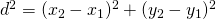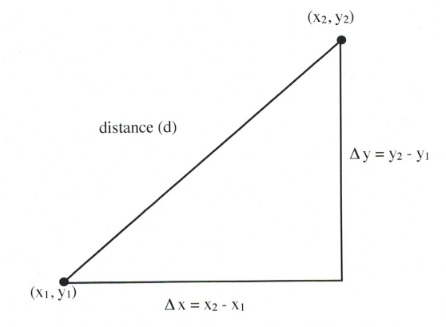On graph paper, this looks like the following. For this illustration, both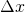and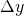are 7 units long, making the distance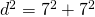or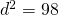.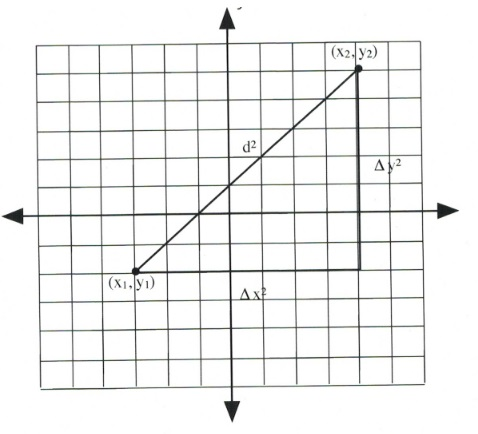The square root of 98 is approximately 9.899 units long.

Example 3.2.1

Find the distance between the points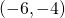and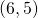.

Start by identifying which are the two data pointsand. Letbeandbe.

Now: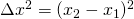orand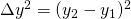or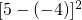.

This means that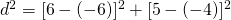or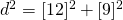which reduces to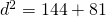or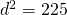Taking the square root, the result is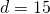.

# Finding the Midway Between Two Points (Midpoint)

The logic used to find the midpoint between two data pointsandon a graph involves finding the average values of the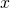data points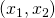and the of the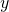data points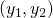. The averages are found by adding both data points together and dividing them by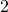.

In an equation, this looks like: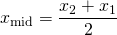and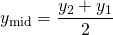Example 3.2.2

Find the midpoint between the points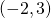and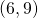.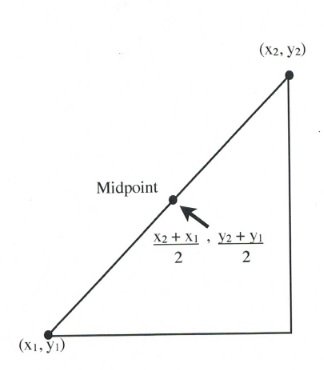We start by adding the twodata points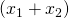and then dividing this result by 2.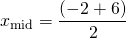or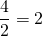The midpoint’s-coordinate is found by adding the twodata points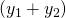and then dividing this result by 2.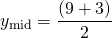or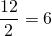The midpoint between the pointsandis at the data point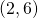.

# Questions

For questions 1 to 8, find the distance between the points.

1.  (−6, −1) and (6, 4)
2. (1, −4) and (5, −1)
3. (−5, −1) and (3, 5)
4. (6, −4) and (12, 4)
5. (−8, −2) and (4, 3)
6. (3, −2) and (7, 1)
7. (−10, −6) and (−2, 0)
8. (8, −2) and (14, 6)

For questions 9 to 16, find the midpoint between the points.

1. (−6, −1) and (6, 5)
2. (1, −4) and (5, −2)
3. (−5, −1) and (3, 5)
4. (6, −4) and (12, 4)
5. (−8, −1) and (6, 7)
6. (1, −6) and (3, −2)
7. (−7, −1) and (3, 9)
8. (2, −2) and (12, 4)